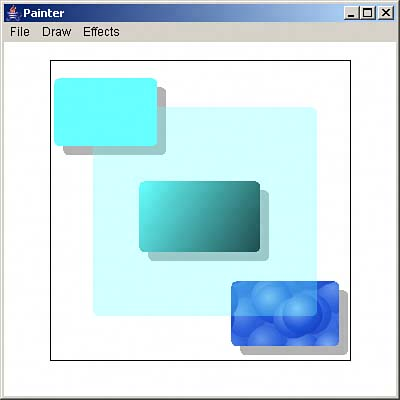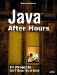Drawing Rounded Rectangles

Another type of figure you can draw using the Graphics2D methods is the rounded rectangle, where the corners are rounded. If the user wants to draw rounded rectangles, you can use the RoundRectangle2D.Double class, whose constructor is just like the Rectangle2D.Double class, except that it also takes two values giving the X and Y radius you want to use for rounding the corners. Painter sets those radii to 10 pixels:

if(rounded && width != 0 && height != 0){     RoundRectangle2D.Double round =         new RoundRectangle2D.Double(tempStart.x,         tempStart.y, width, height, 10, 10); . . .

After you've created the figure you want to draw, you can apply various effects as before, if required:

if(shadow){         paint = gImage.getPaint();         composite = gImage.getComposite();         gImage.setPaint(Color.black);         gImage.setComposite(AlphaComposite.getInstance             (AlphaComposite.SRC_OVER, 0.3f));         RoundRectangle2D.Double round2 =             new RoundRectangle2D.Double(tempStart.x + 9,             tempStart.y + 9, width, height, 10, 10);         if(solid || shade || transparent || texture){             gImage.fill(round2);         }         else{             gImage.draw(round2);         }         gImage.setPaint(paint);         gImage.setComposite(composite);     }     if(solid){         gImage.setPaint(color);     }     if(shade){         gImage.setPaint(             new GradientPaint(                 tempStart.x, tempStart.y, color,                 tempEnd.x, tempEnd.y, Color.black));     }     if(transparent){         gImage.setComposite(AlphaComposite.getInstance         (AlphaComposite.SRC_OVER,0.3f));     }     if(texture){         Rectangle2D.Double anchor =             new Rectangle2D.Double(0,0,             tileImage.getWidth(), tileImage.getHeight());         TexturePaint texturePaint =             new TexturePaint(tileImage,anchor);         gImage.setPaint(texturePaint);     }     if(solid || shade || texture || transparent){         gImage.fill(round);     }     else{         gImage.draw(round);     }     if(transparent){         gImage.setComposite(composite);     } }

That's all it takes; you can see what a variety of rounded rectangles looks like, with some graphics effects added, in Figure 4.5.

Figure 4.5. Various graphics effects with rounded rectangles.Java After Hours: 10 Projects Youll Never Do at Work
ISBN: 0672327473
EAN: 2147483647
Year: 2006
Pages: 128

Similar book on Amazon